# 14.7.5 Astrophysical Parameter Validation: GSP-Spec

## Kiel diagram. Comparison GSP-Phot-GSP-Spec.

In this test we compare the GSP-Phot and GSP-Spec $T_{\rm eff}$ and $\log g$ parameters determined for stellar members of open clusters. This check is carried out on 1508 stellar members of 480 open clusters identified by Cantat-Gaudin et al. (2020). We consider only the sources with the following GSP-Spec flags: FlagVsiniT==0 $\&$ FlagVsiniG==0 $\&$ FlagVsiniM==0 $\&$ FlagVradT==0 $\&$ FlagVradG==0 $\&$ FlagVradM==0 $\&$ FlagNoise==0 $\&$ FlagExtraP$<$=2 $\&$ FlagNegFlux==0 $\&$ FlagNaNFlux==0 $\&$ FlagEmiss==0 $\&$ FlagNullUnc==0 $\&$ FlagKM$<$=0.

The resulting Kiel diagram is shown in Figure 14.96. The sample is predominantly composed by giants, whose sequence is clearly visible at $\log g$ values lower than 3.5 dex. Within this regime there is a good overlap between the GSP-Phot and GSP-Spec stellar parameters.

The sample also contains dwarf stars, i.e., those with $\log g$ values larger than 3.5 dex. In this case GSP-Phot and GSP-Spec overlap only for the few sources hotter than 6000 K. Instead a systematic shift in $\log g$ is clearly visible for stars with lower temperatures. While the GSP-Spec $\log g$ values that are flattened at $\sim$5 dex, the values provided by GSP-Phot are $\sim$4.5 dex, which is closer to the expected values for this type of objects in the main-sequence.

In Figure 14.97 we check the differences in stellar parameters determined by GSP-Phot and GSP-Spec. In the first panel we see that teff_gspphot is typically greater than teff_gspspec for teff_gspspec$<$4500 K. Instead there is a good agreement between GSP-Phot and GSP-Spec for the hotter stars. The middle panel shows a trend indicating that GSP-Phot overestimates the $\log g$ values respect with GSP-Spec. The difference between GSP-Phot and GSP-Spec increases as we move towards lower $\log g$ values. Finally, the bottom panel compares the $[\rm M/H]$ values from GSP-Phot and GSP-Spec. Also for this parameter we observe that at low metallicities GSP-Phot overestimates with respect GSP-Spec.

Finally, in Figure 14.98 we compare the difference in $T_{\rm eff}$ resulting from GSP-Phot and GSP-Spec determinations to the difference in $\log g$. We differentiate between dwarfs (in blue) and giants (in orange), as we observe two distinct behaviours for the two types of stars. Namely, for giants we observe that when GSP-Phot overestimates $T_{\rm eff}$ with respect to GSP-Spec, also $\log g$ is overestimated. Instead, the variations in the two parameters seem uncorrelated for dwarfs. It is well known that the atmospheric parameters are related to each other such that the variation of one of them could lead to the variation of the others. However, the fact that giants and dwarfs are sensitive in different ways to the variation of $T_{\rm eff}$ and $\log g$ could be the result of correlations in the grid of models used for the analysis.Figure 14.96: Kiel diagram for the 1508 sources with high-quality GSP-Spec parameters. In this plot we compare the Teff and log⁡g values derived by GSP-Phot and GSP-Spec.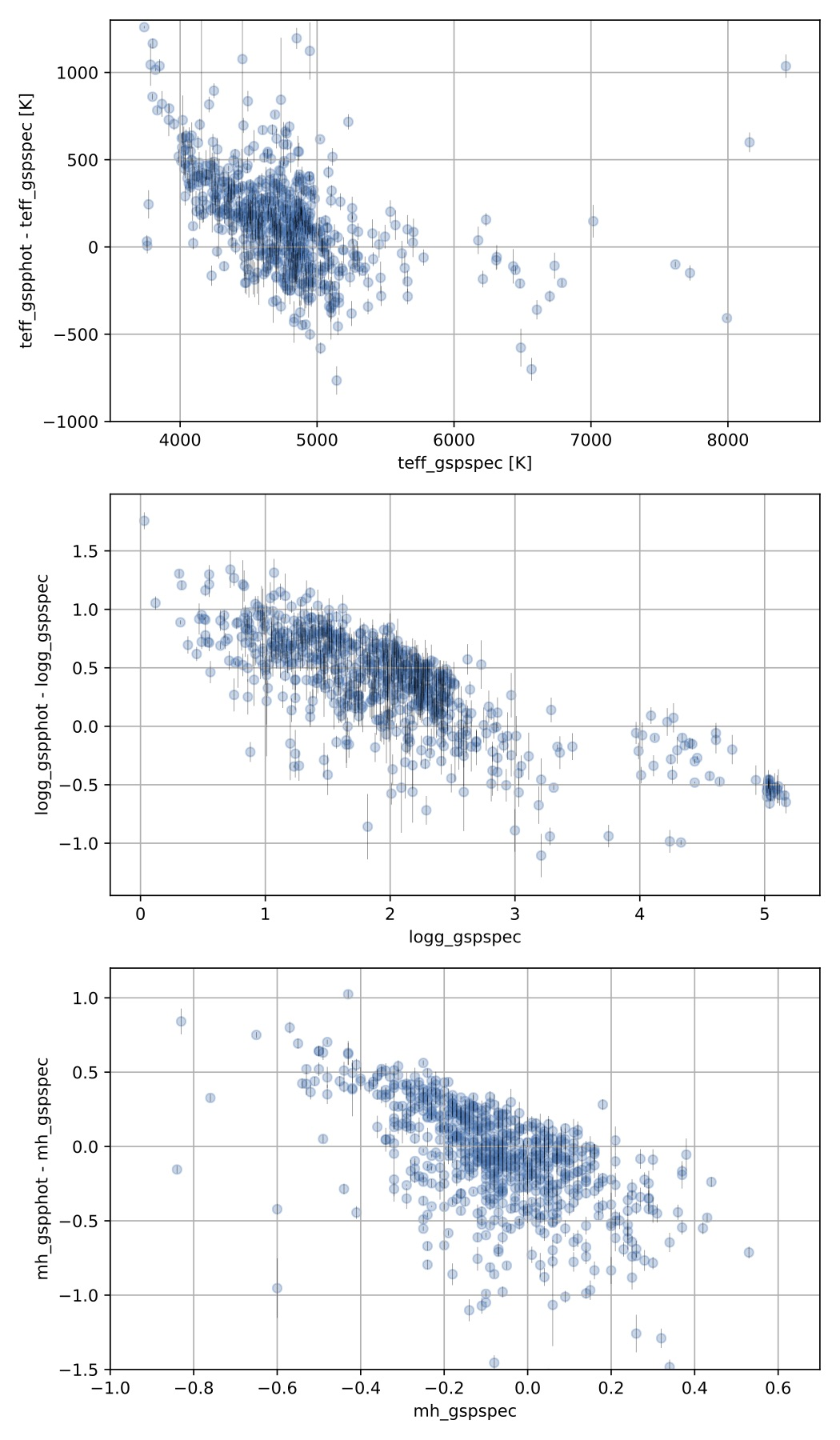Figure 14.97: The three panels show the differences in the stellar parameters Teff, log⁡g, and [M/H] determined by GSP-Phot and GSP-Spec.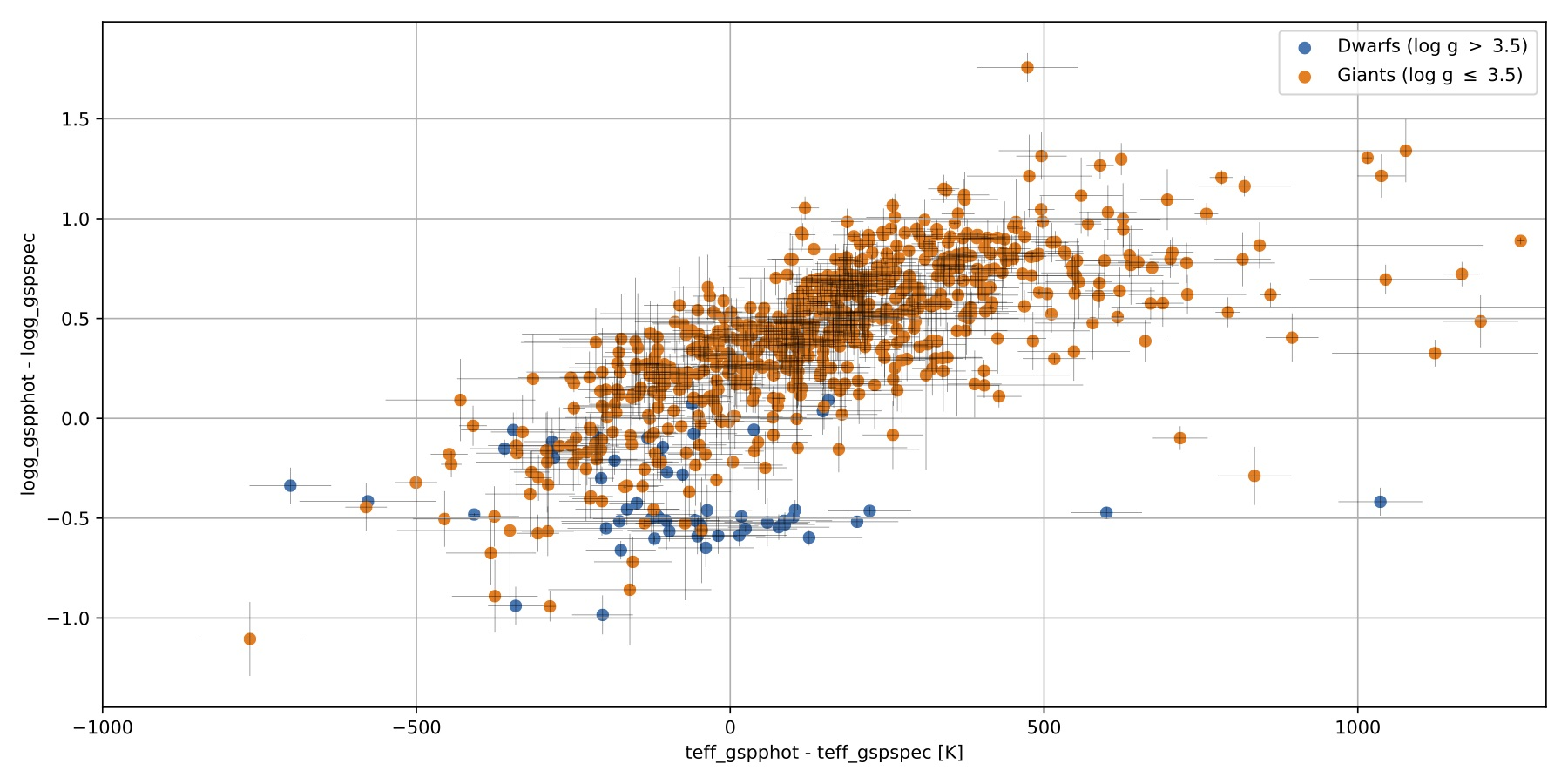Figure 14.98: Difference in log⁡g values derived from GSP-Phot and GSP-Spec as a function of the difference in Teff. Blue points are dwarf stars (log⁡g<3.5 dex), while giants are the orange points.

## Abundances trends in open clusters

Here we checked whether correlations exist between the abundances, the stellar parameters teff_gspspec and logg_gspspec, and the quality parameter logchisq_gspspec. The test sample is composed by stellar members of the open clusters NGC 2477, NGC 2632, NGC 2682, NGC 7789, and Ruprecht 147. We consider only the sources with the following GSP-Spec flags: FlagVsiniT==0 $\&$ FlagVsiniG==0 $\&$ FlagVsiniM==0 $\&$ FlagVradT==0 $\&$ FlagVradG==0 $\&$ FlagVradM==0 $\&$ FlagNoise==0 $\&$ FlagExtraP$<$=2 $\&$ FlagNegFlux==0 $\&$ FlagNaNFlux==0 $\&$ FlagEmiss==0 $\&$ FlagNullUnc==0 $\&$ FlagKM$<$=0.

The panels in Figure 14.99 show the mh_gspspec as a function of teff_gspspec (left column) and logg_gspspec (right column) for each of the five open clusters. Points are colour coded as a function of logchisq_gspspec. The plots show neat positive trends between mh_gspspec and the two stellar parameters teff_gspspec and logg_gspspec. The only exception is NGC 2632, whose stars with teff_gspspec$\geq$6500 K have both low logchisq_gspspec and mh_gspspec values. That strongly indicates that metallicity determination is also affected by the goodness of spectroscopic fit. We also note that the spectra with lowest logchisq_gspspec do not always ensure the best matching with the literature values.

Figure 14.100 shows diagrams similar to those of Figure 14.99 for alphafe_gspspec. Also here we observe trends with the stellar parameters and logchisq_gspspec, however the sign of these relations is not univocal. In fact, while in NGC 2477, NGC 2682, and NGC 7789 show negative trends between the alphafe_gspspec and the stellar parameters, these trends appear to be positive in NGC 2632 and Ruprecht 147.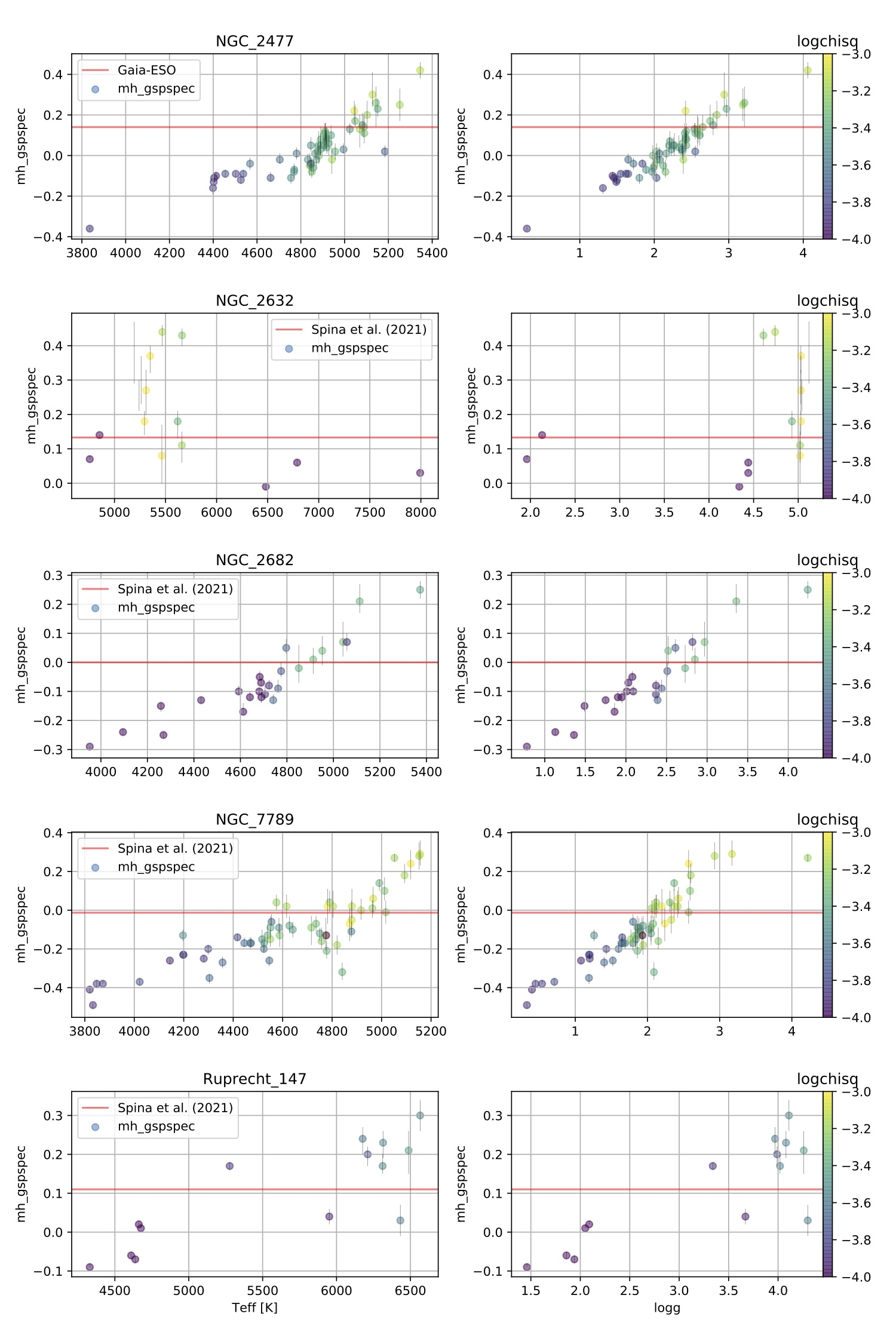Figure 14.99: The panels show the mh_gspspec-teff_gspspec (left column) and mh_gspspec-logg_gspspec diagrams (right column) for the five open clusters NGC 2477, NGC 2632, NGC 2682, NGC 7789, and Ruprecht 147. Symbols are colour coded as a function of logchisq_gspspec.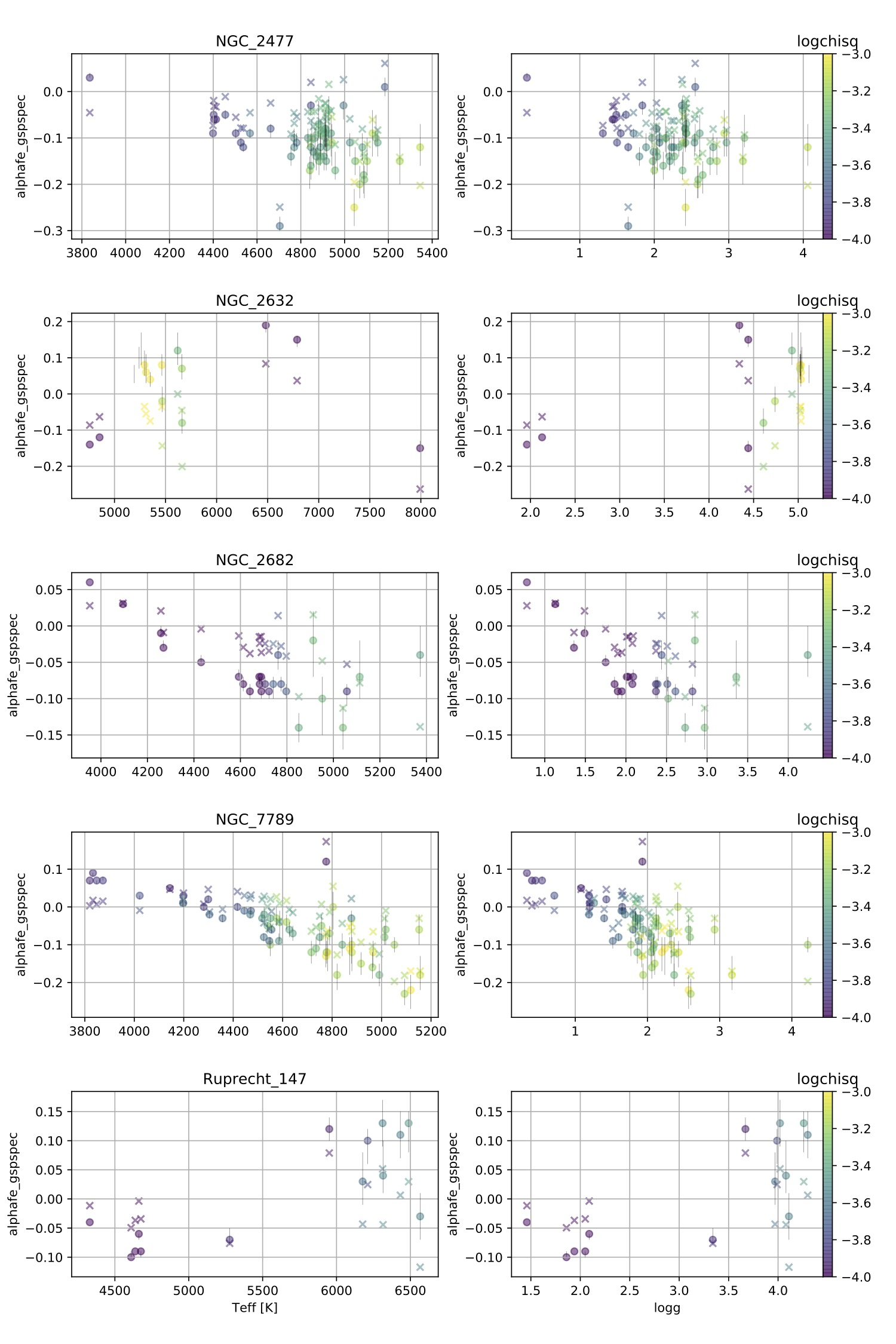Figure 14.100: The panels show the alphafe_gspspec-teff_gspspec (left column) and alphafe_gspspec-logg_gspspec diagrams (right column) for the five open clusters NGC 2477, NGC 2632, NGC 2682, NGC 7789, and Ruprecht 147. Symbols are colour coded as a function of logchisq_gspspec. Circles indicate the uncorrected alphafe_gspspec values taken from Gaia DR3 int5, while crosses represent the calibrated [α/Fe] values.

## Abundance trends in globular clusters

Here we focus on the abundance trends observed in globular clusters, which are stellar populations of much lover metallicity than that of open clusters. In particular we considered the stellar members of NGC 104 (alias 47 Tucani) and NGC 6121 (alias M4) identified by Vasiliev and Baumgardt (2021). We are considering only the sources with the following GSP-Spec flags: FlagVsiniT==0 $\&$ FlagVsiniG==0 $\&$ FlagVsiniM==0 $\&$ FlagVradT==0 $\&$ FlagVradG==0 $\&$ FlagVradM==0 $\&$ FlagNoise==0 $\&$ FlagExtraP$<$=2 $\&$ FlagNegFlux==0 $\&$ FlagNaNFlux==0 $\&$ FlagEmiss==0 $\&$ FlagNullUnc==0 $\&$ FlagKM$<$=0.

Similarly to what we observe for open clusters, also for globular clusters we see neat trends between mh_gspspec, teff_gspspec, logg_gspspec, and logchisq_gspspec (see Figure 14.101 and Figure 14.102).The mh_gspspec values are in agreement with those from the literature. However, negative trends are visible in Figure 14.102 for alphafe_gspspec. Furthermore, there is a discrepancy between the measured alphafe_gspspec values and those of the literature.Figure 14.101: The panels show the mh_gspspec-teff_gspspec (left column) and mh_gspspec-logg_gspspec diagrams (right column) for two globular clusters NGC 104 and NGC 6121. Symbols are colour coded as a function of logchisq_gspspec.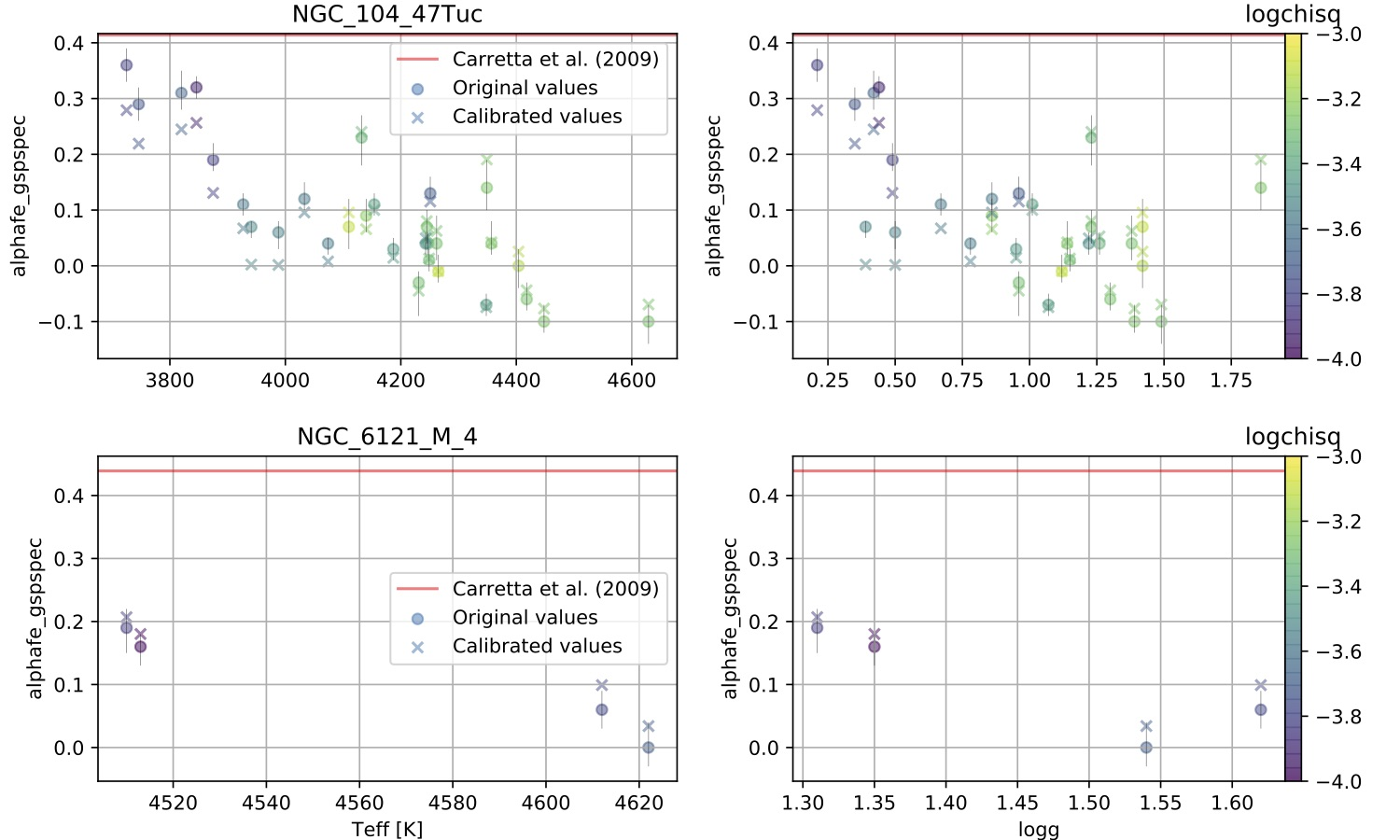Figure 14.102: The panels show the alphafe_gspspec-teff_gspspec (left column) and alphafe_gspspec-logg_gspspec diagrams (right column) for two globular clusters NGC 104 and NGC 6121. Symbols are colour coded as a function of logchisq_gspspec. Circles indicate the uncorrected alphafe_gspspec values taken from Gaia DR3 int5, while crosses represent the calibrated [α/Fe] values.

## Inter- and intra-cluster abundance difference distributions

Stellar members of the same open clusters share the same chemical pattern. Here we test if the observed scatter among abundance determinations within the same cluster can be explained by the abundance uncertainties. We also test if chemical abundances of two stars belonging to the same open cluster are more similar than those of two stars from different clusters. In order to do this we define an intra- and inter-cluster abundance difference normalised for its uncertainty:

 $\hat{\Delta}_{ij}=\frac{A_{i}-A_{j}}{\sqrt{\sigma_{i}^{2}+\sigma_{j}^{2}}},$ (14.33)

where $A_{i}$ and $A_{j}$ are the abundances of the same element derived for the $i^{\rm th}$-star and the $j^{\rm th}$-star, and $\sigma_{i}$ and $\sigma_{j}$ are the uncertainties associated with $A_{i}$ and $A_{j}$. Note that the abundance uncertainties used in this analysis are defined as the half difference between the upper and lower limits. When this difference is equal to zero, we assume an uncertainty equal to 0.01 dex. We also impose that for an intra-cluster $\Delta_{ij}$ the $i^{\rm th}$-star and the $j^{\rm th}$-star must belong to the same cluster, while for the inter-cluster $\Delta_{ij}$ the two stars must belong to different clusters. For this test we considered the cluster members listed in Cantat-Gaudin et al. (2020) with GSP-Spec parameters. Furthermore, we only selected the stars with the following flags: FlagVsiniT==0 $\&$ FlagVsiniG==0 $\&$ FlagVsiniM==0 $\&$ FlagVradT==0 $\&$ FlagVradG==0 $\&$ FlagVradM==0 $\&$ FlagNoise==0 $\&$ FlagExtraP$<$=2 $\&$ FlagNegFlux==0 $\&$ FlagNaNFlux==0 $\&$ FlagEmiss==0 $\&$ FlagNullUnc==0 $\&$ FlagKM$<$=0. Also, when analysing a specific abundance ratio we have also set the two associated flags to that to $<=$1. Finally, we have repeated the experiment on two samples whose stars are selected based on the goodness of their fit: the first sample includes only stars with logchisq_gspspec$<-2.5$, while the second - which is more controlled - includes only high-quality data from stars with logchisq_gspspec$<-3.5$.

The elements that we have explored in this test are mh_gspspec, alphafe_gspspec, fem_gspspec, sife_gspspec, cafe_gspspec, tife_gspspec, nfe_gspspec, and nife_gspspec. In addition to these elements, we also tested the calibrated alphafe_gspspec.

The cumulative distributions of the inter- and intra-clusters normalised abundance differences are shown in Figure 14.103. In addition to the curves described above, in each panel we also plot a ‘reference’ distribution, which represents the case where the abundance scatter is entirely due to the uncertainties in abundance determinations. Therefore, for those elements where the intra-cluster distributions lie below the reference curve, we can conclude that the relative uncertainties are underestimated. In fact, these distributions span normalised differential abundances that are typically larger than expected. This is the case for mh_gspspec, alphafe_gspspec, and cafe_gspspec. Interestingly, we notice that restricting our sample to only those stars with logchisq_gspspec$<-3.5$ - although the inter-cluster scatter is reduced the relative uncertainties result even more underestimated.

Conversely, the uncertainties of all the other elements (i.e., fem_gspspec, sife_gspspec, tife_gspspec, nfe_gspspec, and nife_gspspec) are sightly overestimated. There is no significant difference between the samples selected with the two logchisq_gspspec threshold values.

In Figure 14.103 we also notice that the intra- and inter-cluster curves for sife_gspspec and nfe_gspspec are very similar. For [N/Fe] the effect could be explained by nucleosynthetic processes that can change the abundance of N in stars as they evolve. Instead, the cumulative distributions observed by sife_gspspec suggest that these abundance determinations are highly affected by noise.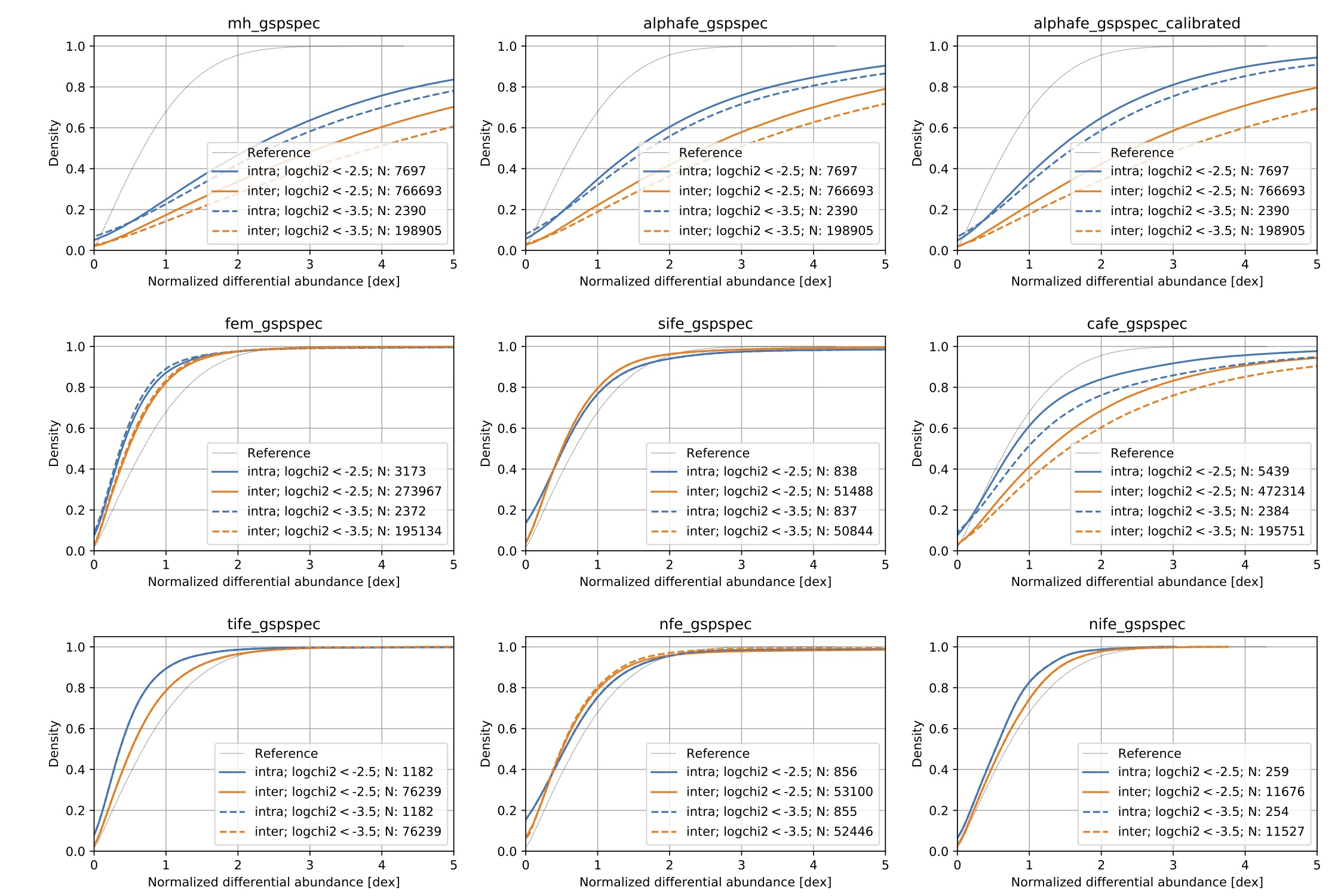Figure 14.103: The distributions have been derived for two samples. The first sample is more inclusive as it comprises all stars with logchisq_gspspec<-2.5. The second is more controlled and only includes stars with logchisq_gspspec<-3.5. The grey line represents the case where the abundance scatter is entirely due to the uncertainties in abundance determinations. This curve serves as a reference to indicate whether the uncertainties of a specific element are typically underestimated or overestimated.